# ILP Part 5 – Absolute value, maximum, minimum

This is the fifth part of the ILP series. For your convenience you can find other parts in the table of contents in Part 1 – Boolean algebra

Last time we defined formulas to compare values. Today we are going to use them to calculate absolute value, maximum, and minimum functions.

# Absolute value

We will start with absolute value. Just like in previous part, we need to know the maximum possible value of a variable denoted as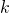.

## Variables

We want to use the following variables:Basically, we have one variable called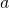. We don’t know whether this variable is positive or negative. We also want to declare variable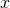which will be equal to absolute value of. So for non-negativeit will be equal to, however, for negativeit will have its value negated.

## Intuition and final formula

We will start with two simple constraints. We wantto be absolute value of, so we know for sure thatmust be: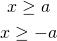This can be interpreted as: “must be on the right side of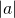on the number line. However, this is not enough becausemight not be equal to.
Now we can use geometric interpretation of absolute value of a number. It is a number’s distance from origin. We wantto have the same distance and to achieve this we need to add the following constraints: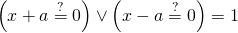This line basically says: either the sum ofandis zero or the sum of negatedandis zero. Here we use relational operators defined in previous part and this is the place where we need to know the upper bound of‘s value.

# Maximum, minimum

Using similar intuition we can define maximum and minimum functions. We start with the following variables: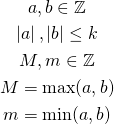First, we wantto be “on the right side” ofand: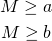Similarly, we want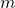to be “on the left side”: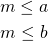And now the final piece: we want the distance betweenandto be the equal to the distance betweenand:We need to use the absolute value only on the left side because we know that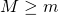.
However, this will not work. In previous part we defined and used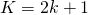and we showed why it is important to define it this way. However, our absolute value function uses relational operators, and these assume that their arguments are not greater than. But for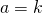and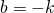we break this constraint. There are two possible solutions:

## Solution 1

We can change the definition of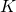to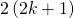. This will work but it has one drawback: imagine that our solver is able to perform calculations on 32-bit integers. Because we need to be able to representusing 32 bits, we are effectively reducing the size of the rest of our variables. This might be painful.

## Solution 2

We might want to change the last constraint to: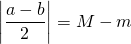In theory, this should work. However, imagine the situation when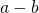is odd. We need to represent the result of division using floating point values and this might cause some problems with representation errors with more sophisticated calculations. However, using this approach we do not reduce the size of our variables as with the previous solution.

# Summary

We have defined three useful functions. In next parts we will see that they might look trivial, but they are really powerful and we will use them to define much more complicated calculations. See you next time.